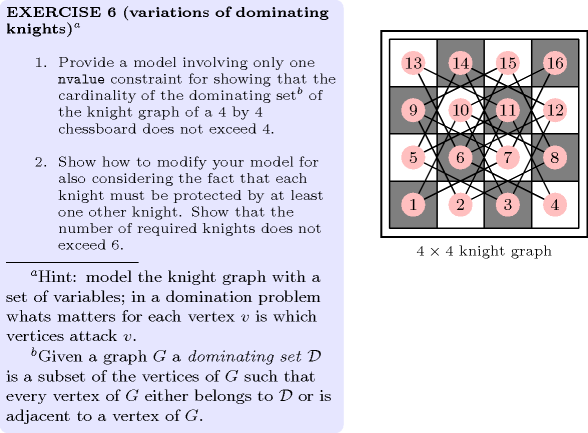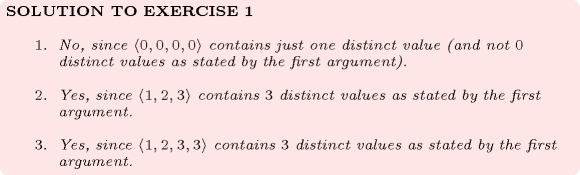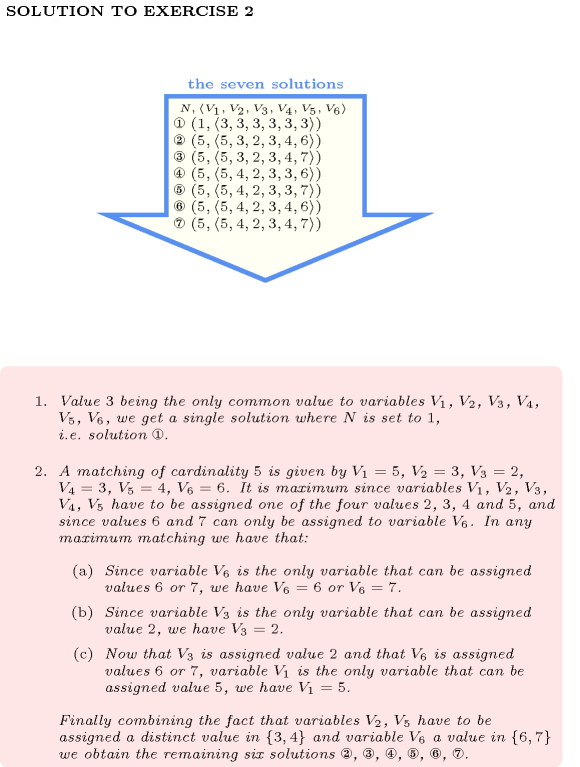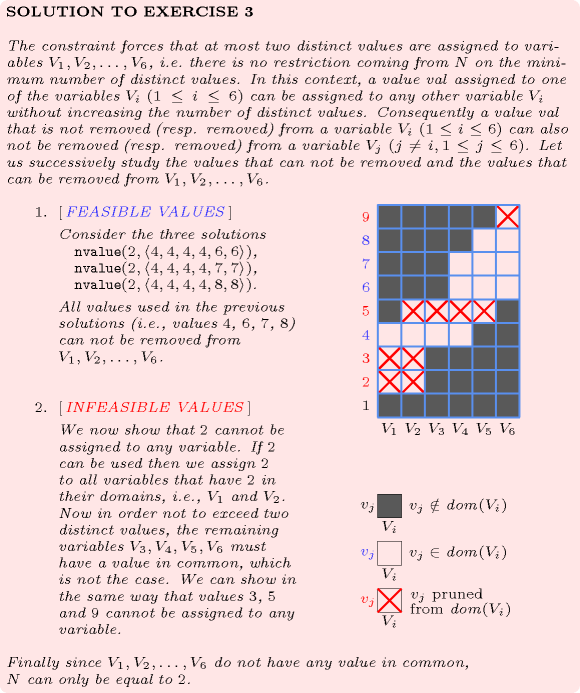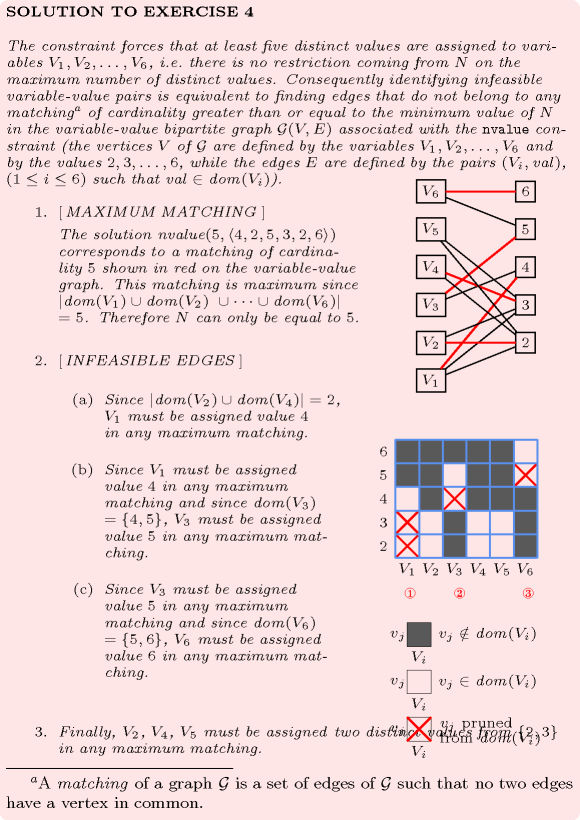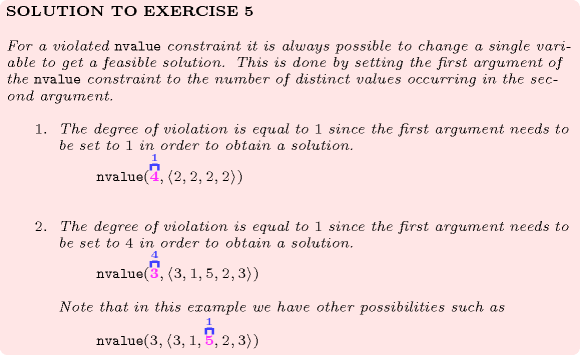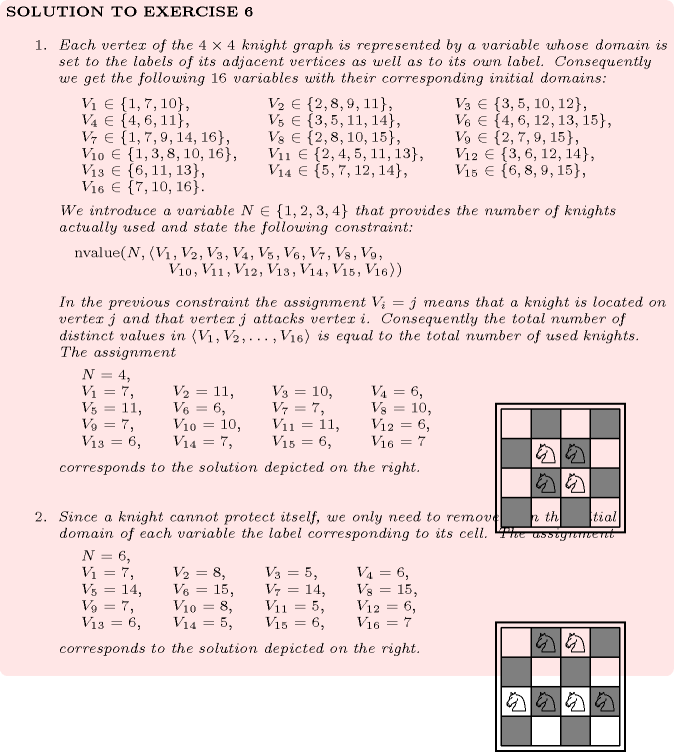## 5.286. nvalue

Origin
Constraint

$\mathrm{𝚗𝚟𝚊𝚕𝚞𝚎}\left(\mathrm{𝙽𝚅𝙰𝙻},\mathrm{𝚅𝙰𝚁𝙸𝙰𝙱𝙻𝙴𝚂}\right)$

Synonyms

$\mathrm{𝚌𝚊𝚛𝚍𝚒𝚗𝚊𝚕𝚒𝚝𝚢}_\mathrm{𝚘𝚗}_\mathrm{𝚊𝚝𝚝𝚛𝚒𝚋𝚞𝚝𝚎𝚜}_\mathrm{𝚟𝚊𝚕𝚞𝚎𝚜}$, $\mathrm{𝚟𝚊𝚕𝚞𝚎𝚜}$.

Arguments
 $\mathrm{𝙽𝚅𝙰𝙻}$ $\mathrm{𝚍𝚟𝚊𝚛}$ $\mathrm{𝚅𝙰𝚁𝙸𝙰𝙱𝙻𝙴𝚂}$ $\mathrm{𝚌𝚘𝚕𝚕𝚎𝚌𝚝𝚒𝚘𝚗}\left(\mathrm{𝚟𝚊𝚛}-\mathrm{𝚍𝚟𝚊𝚛}\right)$
Restrictions
 $\mathrm{𝚛𝚎𝚚𝚞𝚒𝚛𝚎𝚍}$$\left(\mathrm{𝚅𝙰𝚁𝙸𝙰𝙱𝙻𝙴𝚂},\mathrm{𝚟𝚊𝚛}\right)$ $\mathrm{𝙽𝚅𝙰𝙻}\ge \mathrm{𝚖𝚒𝚗}\left(1,|\mathrm{𝚅𝙰𝚁𝙸𝙰𝙱𝙻𝙴𝚂}|\right)$ $\mathrm{𝙽𝚅𝙰𝙻}\le |\mathrm{𝚅𝙰𝚁𝙸𝙰𝙱𝙻𝙴𝚂}|$ $\mathrm{𝙽𝚅𝙰𝙻}\le$$\mathrm{𝚛𝚊𝚗𝚐𝚎}$$\left(\mathrm{𝚅𝙰𝚁𝙸𝙰𝙱𝙻𝙴𝚂}.\mathrm{𝚟𝚊𝚛}\right)$
Purpose

$\mathrm{𝙽𝚅𝙰𝙻}$ is the number of distinct values taken by the variables of the collection $\mathrm{𝚅𝙰𝚁𝙸𝙰𝙱𝙻𝙴𝚂}$.

Example
 $\left(4,〈3,1,7,1,6〉\right)$ $\left(1,〈6,6,6,6,6〉\right)$ $\left(5,〈6,3,0,2,9〉\right)$
• The first $\mathrm{𝚗𝚟𝚊𝚕𝚞𝚎}$ constraint holds since its first argument $\mathrm{𝙽𝚅𝙰𝙻}=4$ is set to the number of distinct values occurring within the collection $〈3,1,7,1,6〉$.

• The second $\mathrm{𝚗𝚟𝚊𝚕𝚞𝚎}$ constraint holds since its first argument $\mathrm{𝙽𝚅𝙰𝙻}=1$ is set to the number of distinct values occurring within the collection $〈6,6,6,6,6〉$.

• The third $\mathrm{𝚗𝚟𝚊𝚕𝚞𝚎}$ constraint holds since its first argument $\mathrm{𝙽𝚅𝙰𝙻}=5$ is set to the number of distinct values occurring within the collection $〈6,3,0,2,9〉$.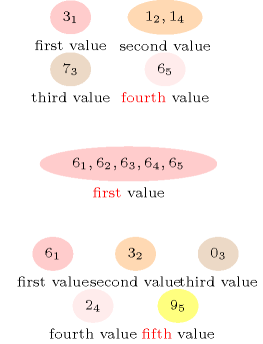All solutions

Figure 5.286.1 gives all solutions to the following non ground instance of the $\mathrm{𝚗𝚟𝚊𝚕𝚞𝚎}$ constraint: $N\in \left[1,2\right]$, ${V}_{1}\in \left[2,4\right]$, ${V}_{2}\in \left[1,2\right]$, ${V}_{3}\in \left[2,4\right]$, $\mathrm{𝚗𝚟𝚊𝚕𝚞𝚎}$$\left(N,〈{V}_{1},{V}_{2},{V}_{3}〉\right)$.

##### Figure 5.286.1. All solutions corresponding to the non ground example of the $\mathrm{𝚗𝚟𝚊𝚕𝚞𝚎}$ constraint of the All solutions slot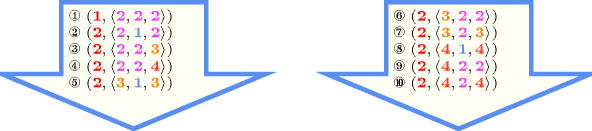Typical
 $\mathrm{𝙽𝚅𝙰𝙻}>1$ $\mathrm{𝙽𝚅𝙰𝙻}<|\mathrm{𝚅𝙰𝚁𝙸𝙰𝙱𝙻𝙴𝚂}|$ $|\mathrm{𝚅𝙰𝚁𝙸𝙰𝙱𝙻𝙴𝚂}|>1$
Symmetries
• Items of $\mathrm{𝚅𝙰𝚁𝙸𝙰𝙱𝙻𝙴𝚂}$ are permutable.

• All occurrences of two distinct values of $\mathrm{𝚅𝙰𝚁𝙸𝙰𝙱𝙻𝙴𝚂}.\mathrm{𝚟𝚊𝚛}$ can be swapped; all occurrences of a value of $\mathrm{𝚅𝙰𝚁𝙸𝙰𝙱𝙻𝙴𝚂}.\mathrm{𝚟𝚊𝚛}$ can be renamed to any unused value.

Arg. properties
• Functional dependency: $\mathrm{𝙽𝚅𝙰𝙻}$ determined by $\mathrm{𝚅𝙰𝚁𝙸𝙰𝙱𝙻𝙴𝚂}$.

• Contractible wrt. $\mathrm{𝚅𝙰𝚁𝙸𝙰𝙱𝙻𝙴𝚂}$ when $\mathrm{𝙽𝚅𝙰𝙻}=1$ and $|\mathrm{𝚅𝙰𝚁𝙸𝙰𝙱𝙻𝙴𝚂}|>0$.

• Contractible wrt. $\mathrm{𝚅𝙰𝚁𝙸𝙰𝙱𝙻𝙴𝚂}$ when $\mathrm{𝙽𝚅𝙰𝙻}=|\mathrm{𝚅𝙰𝚁𝙸𝙰𝙱𝙻𝙴𝚂}|$.

Usage

The $\mathrm{𝚗𝚟𝚊𝚕𝚞𝚎}$ constraint allows relaxing the $\mathrm{𝚊𝚕𝚕𝚍𝚒𝚏𝚏𝚎𝚛𝚎𝚗𝚝}$ constraint by restricting its first argument $\mathrm{𝙽𝚅𝙰𝙻}$ to be close, but not necessarily equal, to the number of variables of the $\mathrm{𝚅𝙰𝚁𝙸𝙰𝙱𝙻𝙴𝚂}$ collection.

A classical example from the early 1850s is the dominating queens chess puzzle problem: Place a number of queens on an $n$ by $n$ chessboard in such a way that all cells of the chessboard are either attacked by a queen or are occupied by a queen. A queen can attack all cells located on the same column, on the same row or on the same diagonal. Part (A) of Figure 5.286.2 illustrates a set of five queens which together attack all of the cells of an 8 by 8 chessboard. The dominating queens problem can be modelled by just one $\mathrm{𝚗𝚟𝚊𝚕𝚞𝚎}$ constraint:

• We first label the different cells of the chessboard from 1 to ${n}^{2}$.

• We then associate to each cell $c$ of the chessboard a domain variable. Its initial domain is set to the labels of the cells that can attack cell $c$. For instance, in the context of an 8 by 8 chessboard, the initial domain of ${V}_{29}$ will be set to {2,5,8,11,13,15,20..22,25..32,36..38,43,45,47,50,53,56,57,61} (see the green cells of part (B) of Figure 5.286.2).

• Finally, we post the constraint $\mathrm{𝚗𝚟𝚊𝚕𝚞𝚎}$$\left(Q,〈\mathrm{𝚟𝚊𝚛}-{V}_{1},\mathrm{𝚟𝚊𝚛}-{V}_{2},\cdots ,\mathrm{𝚟𝚊𝚛}-{V}_{{n}^{2}}〉\right)$ where $Q$ is a domain variable in $\left[1,{n}^{2}\right]$ that gives the total number of queens used for controlling all cells of the chessboard. For the solution depicted by Part (A) of Figure 5.286.2, the label in each cell of Part (C) of Figure 5.286.2 gives the value assigned to the corresponding variable. Note that, since a given cell can be attacked by several queens, we have also other assignments corresponding to the solution depicted by Part (A) of Figure 5.286.2.

##### Figure 5.286.2. Modelling the dominating queens problem with a single $\mathrm{𝚗𝚟𝚊𝚕𝚞𝚎}$ constraint; (A) a solution to the dominating queens problem, (B) the initial domain (in bold) of the variable associated with cell 29: in a solution the value $j$ assigned to the variable associated with cell $i$ represents the label of the cell attacking cell $i$ (i.e. in a solution one of the selected queens is located on cell $j$), (C) the value of each cell in the model with one single $\mathrm{𝚗𝚟𝚊𝚕𝚞𝚎}$ constraint corresponding to the solution depicted in (A).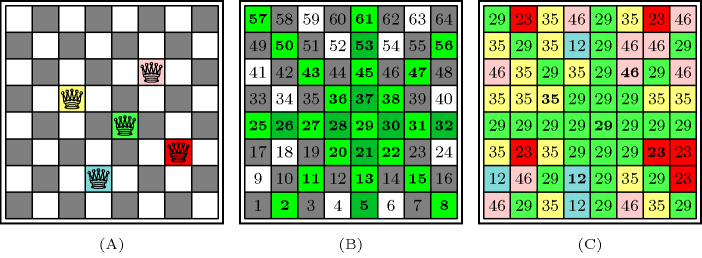The $\mathrm{𝚗𝚟𝚊𝚕𝚞𝚎}$ constraint occurs also in many practical applications. In the context of timetabling one wants to set up a limit on the maximum number of activity types it is possible to perform. For frequency allocation problems, one optimisation criterion is to minimise the number of distinct frequencies that you use all over the entire network.

The $\mathrm{𝚗𝚟𝚊𝚕𝚞𝚎}$ constraint generalises several constraints like:

Remark

This constraint appears in [PachetRoy99] under the name of Cardinality on Attributes Values. The $\mathrm{𝚗𝚟𝚊𝚕𝚞𝚎}$ constraint is called $\mathrm{𝚟𝚊𝚕𝚞𝚎𝚜}$ in JaCoP (http://www.jacop.eu/). A constraint called $𝚔_\mathrm{𝚍𝚒𝚏𝚏}$ enforcing that a set of variables takes at least $k$ distinct values appears in the PhD thesis of J.-C. Régin [Regin95].

It was shown in [BessiereHebrardHnichWalshO4] that, finding out whether a $\mathrm{𝚗𝚟𝚊𝚕𝚞𝚎}$ constraint has a solution or not is NP-hard. This was achieved by reduction from 3-SAT. In the same article, it is also shown, by reduction from minimum hitting set cardinality, that computing a sharp lower bound on $\mathrm{𝙽𝚅𝙰𝙻}$ is NP-hard.

Both reformulations of the $\mathrm{𝚌𝚘𝚕𝚘𝚞𝚛𝚎𝚍}_\mathrm{𝚌𝚞𝚖𝚞𝚕𝚊𝚝𝚒𝚟𝚎}$ constraint and of the $\mathrm{𝚌𝚘𝚕𝚘𝚞𝚛𝚎𝚍}_\mathrm{𝚌𝚞𝚖𝚞𝚕𝚊𝚝𝚒𝚟𝚎𝚜}$ constraint use the $\mathrm{𝚗𝚟𝚊𝚕𝚞𝚎}$ constraint.

Algorithm

A first filtering algorithm for the $\mathrm{𝚗𝚟𝚊𝚕𝚞𝚎}$ constraint was described in [Beldiceanu01]. Assuming that the minimum value of variable $\mathrm{𝙽𝚅𝙰𝙻}$ is not constrained at all, two algorithms that both achieve bound-consistency were provided one year later in [BeldiceanuCarlssonThiel02]. Under the same assumption, algorithms that partially take into account holes in the domains of the variables of the $\mathrm{𝚅𝙰𝚁𝙸𝙰𝙱𝙻𝙴𝚂}$ collection are described in [BeldiceanuCarlssonThiel02], [BessiereHebrardHnichKiziltanWalsh05].

Reformulation

A model, involving linear inequalities constraints, preserving bound-consistency was introduced in [BessiereKatsirelosNarodytskaQuimperWalsh10CP].

Counting
 Length ($n$) 2 3 4 5 6 7 8 Solutions 9 64 625 7776 117649 2097152 43046721

Number of solutions for $\mathrm{𝚗𝚟𝚊𝚕𝚞𝚎}$: domains $0..n$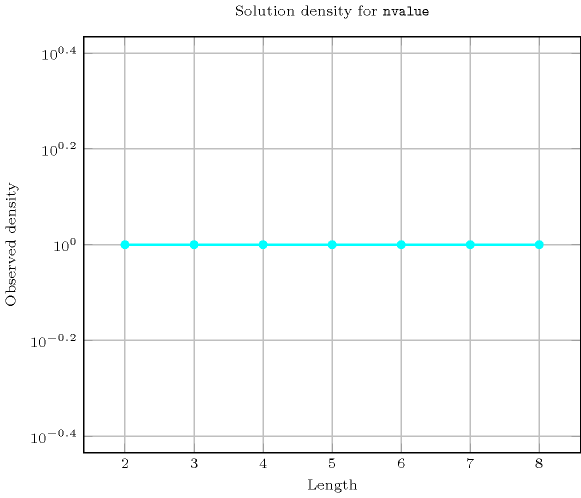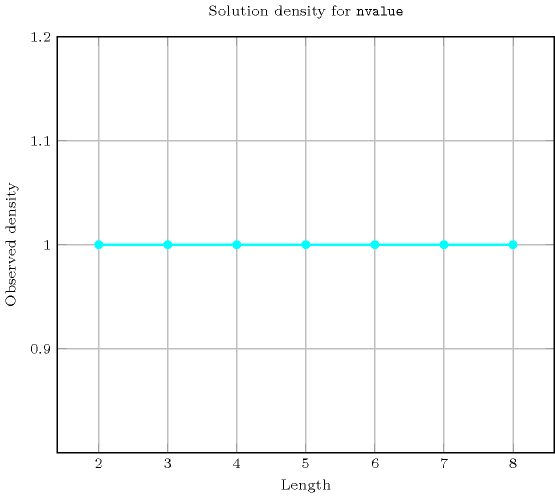Length ($n$)2345678
Total9646257776117649209715243046721
 Parameter value

13456789
2636140450130235289144
3-24360300018900101136486864
4--1203600546005880005143824
5---7203780094080015876000
6----504042336016087680
7-----403205080320
8------362880

Solution count for $\mathrm{𝚗𝚟𝚊𝚕𝚞𝚎}$: domains $0..n$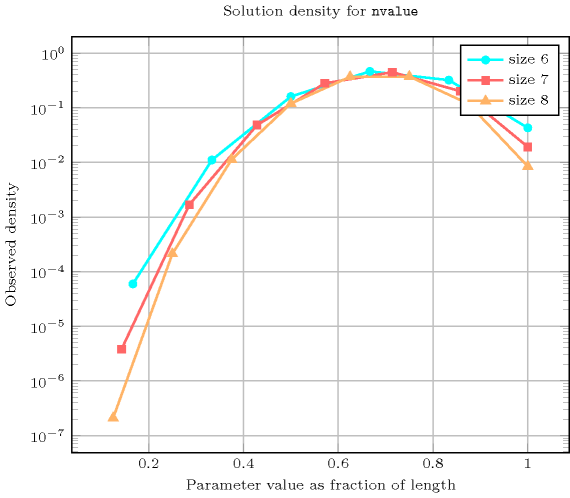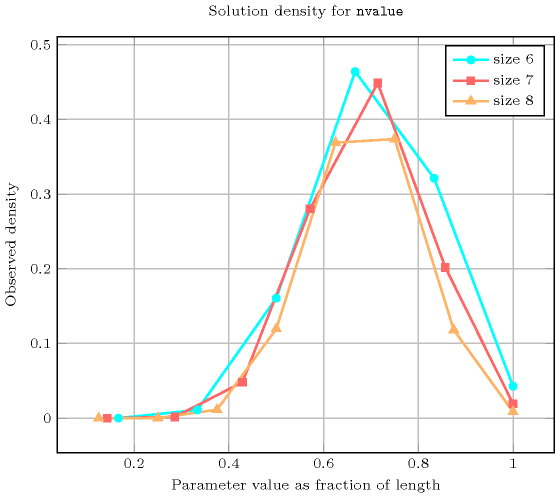Systems
Used in

cost variant: $\mathrm{𝚜𝚞𝚖}_\mathrm{𝚘𝚏}_\mathrm{𝚠𝚎𝚒𝚐𝚑𝚝𝚜}_\mathrm{𝚘𝚏}_\mathrm{𝚍𝚒𝚜𝚝𝚒𝚗𝚌𝚝}_\mathrm{𝚟𝚊𝚕𝚞𝚎𝚜}$ (introduce a weight for each value and replace number of distinct values by sum of weights associated with distinct values).

generalisation: $\mathrm{𝚗𝚌𝚕𝚊𝚜𝚜}$ ($\mathrm{𝚟𝚊𝚛𝚒𝚊𝚋𝚕𝚎}$ replaced by $\mathrm{𝚟𝚊𝚛𝚒𝚊𝚋𝚕𝚎}\in \mathrm{𝚙𝚊𝚛𝚝𝚒𝚝𝚒𝚘𝚗}$), $\mathrm{𝚗𝚎𝚚𝚞𝚒𝚟𝚊𝚕𝚎𝚗𝚌𝚎}$ ($\mathrm{𝚟𝚊𝚛𝚒𝚊𝚋𝚕𝚎}$ replaced by $\mathrm{𝚟𝚊𝚛𝚒𝚊𝚋𝚕𝚎}\mathrm{mod}\mathrm{𝚌𝚘𝚗𝚜𝚝𝚊𝚗𝚝}$), $\mathrm{𝚗𝚒𝚗𝚝𝚎𝚛𝚟𝚊𝚕}$ ($\mathrm{𝚟𝚊𝚛𝚒𝚊𝚋𝚕𝚎}$ replaced by $\mathrm{𝚟𝚊𝚛𝚒𝚊𝚋𝚕𝚎}/\mathrm{𝚌𝚘𝚗𝚜𝚝𝚊𝚗𝚝}$), $\mathrm{𝚗𝚙𝚊𝚒𝚛}$ ($\mathrm{𝚟𝚊𝚛𝚒𝚊𝚋𝚕𝚎}$ replaced by $\mathrm{𝚙𝚊𝚒𝚛}$ of $\mathrm{𝚟𝚊𝚛𝚒𝚊𝚋𝚕𝚎𝚜}$), $\mathrm{𝚗𝚟𝚊𝚕𝚞𝚎𝚜}$ (replace an equality with the number of distinct values by a comparison with the number of distinct values), $\mathrm{𝚗𝚟𝚎𝚌𝚝𝚘𝚛}$ (variable replaced by vector).

implies: $\mathrm{𝚊𝚝𝚕𝚎𝚊𝚜𝚝}_\mathrm{𝚗𝚟𝚊𝚕𝚞𝚎}$ ($=\mathrm{𝙽𝚅𝙰𝙻}$ replaced by $\ge \mathrm{𝙽𝚅𝙰𝙻}$), $\mathrm{𝚊𝚝𝚖𝚘𝚜𝚝}_\mathrm{𝚗𝚟𝚊𝚕𝚞𝚎}$ ($=\mathrm{𝙽𝚅𝙰𝙻}$ replaced by $\le \mathrm{𝙽𝚅𝙰𝙻}$).

related: $\mathrm{𝚋𝚊𝚕𝚊𝚗𝚌𝚎}$ (restriction on how balanced an assignment is), $\mathrm{𝚌𝚘𝚕𝚘𝚞𝚛𝚎𝚍}_\mathrm{𝚌𝚞𝚖𝚞𝚕𝚊𝚝𝚒𝚟𝚎}$ (restrict number of distinct colours on each maximum clique of the interval graph associated with the tasks), $\mathrm{𝚌𝚘𝚕𝚘𝚞𝚛𝚎𝚍}_\mathrm{𝚌𝚞𝚖𝚞𝚕𝚊𝚝𝚒𝚟𝚎𝚜}$ (restrict number of distinct colours on each maximum clique of the interval graph associated with the tasks assigned to the same machine), $\mathrm{𝚒𝚗𝚌𝚛𝚎𝚊𝚜𝚒𝚗𝚐}_\mathrm{𝚗𝚟𝚊𝚕𝚞𝚎}_\mathrm{𝚌𝚑𝚊𝚒𝚗}$, $𝚔_\mathrm{𝚊𝚕𝚕𝚍𝚒𝚏𝚏𝚎𝚛𝚎𝚗𝚝}$ (necessary condition for two overlapping $\mathrm{𝚊𝚕𝚕𝚍𝚒𝚏𝚏𝚎𝚛𝚎𝚗𝚝}$ constraints), $\mathrm{𝚜𝚘𝚏𝚝}_\mathrm{𝚊𝚕𝚕𝚍𝚒𝚏𝚏𝚎𝚛𝚎𝚗𝚝}_\mathrm{𝚟𝚊𝚛}$.

specialisation: $\mathrm{𝚊𝚕𝚕}_\mathrm{𝚎𝚚𝚞𝚊𝚕}$ (enforce to have one single value), $\mathrm{𝚊𝚕𝚕𝚍𝚒𝚏𝚏𝚎𝚛𝚎𝚗𝚝}$ (enforce a number of distinct values equal to the number of variables), $\mathrm{𝚗𝚘𝚝}_\mathrm{𝚊𝚕𝚕}_\mathrm{𝚎𝚚𝚞𝚊𝚕}$ (enforce to have at least two distinct values).

Keywords
Cond. implications

$\mathrm{𝚗𝚟𝚊𝚕𝚞𝚎}\left(\mathrm{𝙽𝚅𝙰𝙻},\mathrm{𝚅𝙰𝚁𝙸𝙰𝙱𝙻𝙴𝚂}\right)$

with  $\mathrm{𝚒𝚗𝚌𝚛𝚎𝚊𝚜𝚒𝚗𝚐}$$\left(\mathrm{𝚅𝙰𝚁𝙸𝙰𝙱𝙻𝙴𝚂}\right)$

implies $\mathrm{𝚒𝚗𝚌𝚛𝚎𝚊𝚜𝚒𝚗𝚐}_\mathrm{𝚗𝚟𝚊𝚕𝚞𝚎}$$\left(\mathrm{𝙽𝚅𝙰𝙻},\mathrm{𝚅𝙰𝚁𝙸𝙰𝙱𝙻𝙴𝚂}\right)$.

Arc input(s)

$\mathrm{𝚅𝙰𝚁𝙸𝙰𝙱𝙻𝙴𝚂}$

Arc generator
$\mathrm{𝐶𝐿𝐼𝑄𝑈𝐸}$$↦\mathrm{𝚌𝚘𝚕𝚕𝚎𝚌𝚝𝚒𝚘𝚗}\left(\mathrm{𝚟𝚊𝚛𝚒𝚊𝚋𝚕𝚎𝚜}\mathtt{1},\mathrm{𝚟𝚊𝚛𝚒𝚊𝚋𝚕𝚎𝚜}\mathtt{2}\right)$

Arc arity
Arc constraint(s)
$\mathrm{𝚟𝚊𝚛𝚒𝚊𝚋𝚕𝚎𝚜}\mathtt{1}.\mathrm{𝚟𝚊𝚛}=\mathrm{𝚟𝚊𝚛𝚒𝚊𝚋𝚕𝚎𝚜}\mathtt{2}.\mathrm{𝚟𝚊𝚛}$
Graph property(ies)
$\mathrm{𝐍𝐒𝐂𝐂}$$=\mathrm{𝙽𝚅𝙰𝙻}$

Graph class
$\mathrm{𝙴𝚀𝚄𝙸𝚅𝙰𝙻𝙴𝙽𝙲𝙴}$

Graph model

Parts (A) and (B) of Figure 5.286.3 respectively show the initial and final graph associated with the first example of the Example slot. Since we use the $\mathrm{𝐍𝐒𝐂𝐂}$ graph property we show the different strongly connected components of the final graph. Each strongly connected component corresponds to a value that is assigned to some variables of the $\mathrm{𝚅𝙰𝚁𝙸𝙰𝙱𝙻𝙴𝚂}$ collection. The 4 following values 1, 3, 6 and 7 are used by the variables of the $\mathrm{𝚅𝙰𝚁𝙸𝙰𝙱𝙻𝙴𝚂}$ collection.

##### Figure 5.286.3. Initial and final graph of the $\mathrm{𝚗𝚟𝚊𝚕𝚞𝚎}$ constraint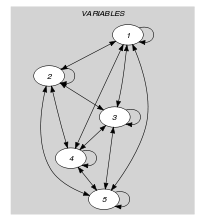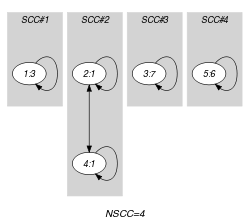(a) (b)
Automaton

Figure 5.286.4 depicts the automaton associated with the $\mathrm{𝚗𝚟𝚊𝚕𝚞𝚎}$ constraint. To each item of the collection $\mathrm{𝚅𝙰𝚁𝙸𝙰𝙱𝙻𝙴𝚂}$ corresponds a signature variable ${S}_{i}$ that is equal to 0.

##### Figure 5.286.4. Automaton of the $\mathrm{𝚗𝚟𝚊𝚕𝚞𝚎}$ constraint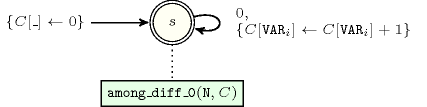Quiz

$\mathrm{𝚗𝚟𝚊𝚕𝚞𝚎}$: checking whether a ground instance holds or not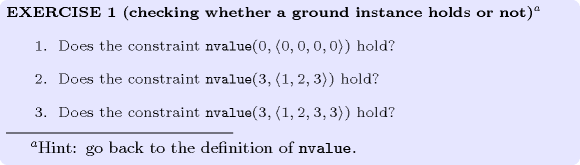$\mathrm{𝚗𝚟𝚊𝚕𝚞𝚎}$: finding all solutions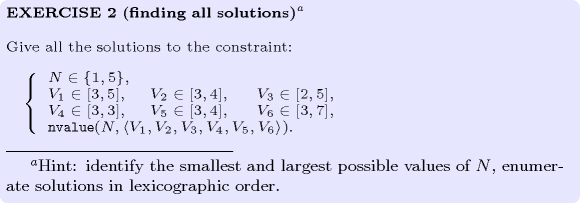$\mathrm{𝚗𝚟𝚊𝚕𝚞𝚎}$: identifying infeasible values wrt the at most side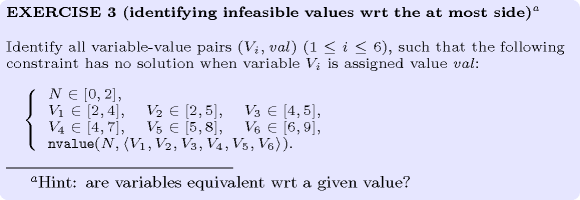$\mathrm{𝚗𝚟𝚊𝚕𝚞𝚎}$: identifying infeasible variable-value pairs wrt the at least side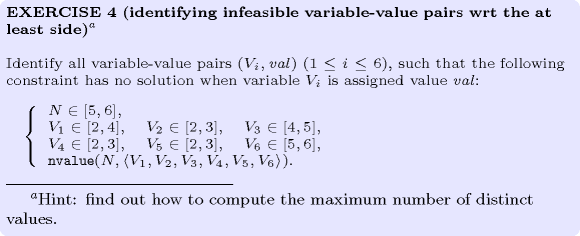$\mathrm{𝚗𝚟𝚊𝚕𝚞𝚎}$: variable-based degree of violation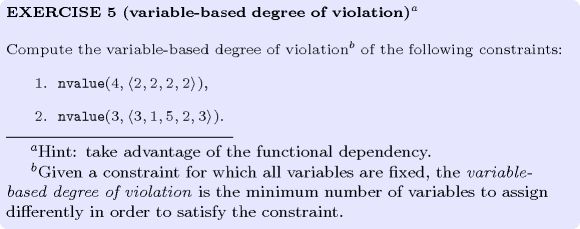$\mathrm{𝚗𝚟𝚊𝚕𝚞𝚎}$: variations of dominating knights